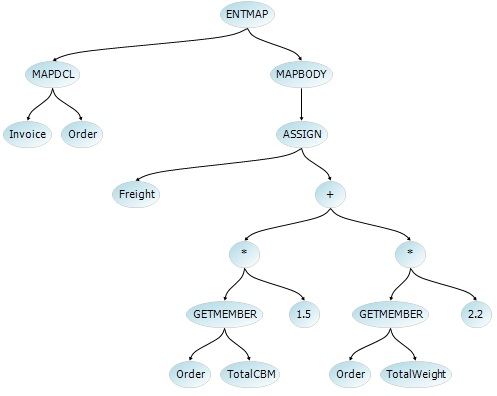# How to write your own custom AST parser?

In Yaydoc, we are using pandoc to convert text from one format to another. Pandoc is one of the best text conversion tool which helps users to convert text between different markup formats. It is written in HASKELL. Many wrapper libraries are available for different programming languages which include python, nodejs, ruby. But in yaydoc, for a few particular scenarios we have to customize the conversion to meet our needs. So I started to build to a custom parser. The parser which I made will convert yml code block to yaml code block because sphinx need yaml code block for rendering. In order to parse, we have to split the text into tokens to our need. So initially we have to write a lexer to split the text into tokens. Here is the sample snippet for a basic lexer.

```class Node:
def __init__(self, text, token):
self.text = text
self.token = token

def __str__(self):
return self.text+' '+self.token

def lexer(text):
def syntax_highliter_lexer(nodes, words):
splitted_syntax_highligter = words.split('```')
if splitted_syntax_highligter is not '':
nodes.append(Node(splitted_syntax_highligter, 'WORD'))
splitted_syntax_highligter = '```'
words = ''.join([x for x in splitted_syntax_highligter])
nodes.append(Node(words, 'SYNTAX HIGHLIGHTER'))
return nodes

syntax_re = re.compile('```')
nodes = []
pos = 0
words = ''
while pos < len(text):
if text[pos] == ' ':
if len(words) > 0:
if syntax_re.search(words) is not None:
nodes = syntax_highliter_lexer(nodes, words)
else:
nodes.append(Node(words, 'WORD'))
words = ''
nodes.append(Node(text[pos], 'SPACE'))
pos = pos + 1
elif text[pos] == '\n':
if len(words) > 0:
if syntax_re.search(words) is not None:
nodes = syntax_highliter_lexer(nodes, words)
else:
nodes.append(Node(words, 'WORD'))
words = ''
nodes.append(Node(text[pos], 'NEWLINE'))
pos = pos + 1
else:
words += text[pos]
pos = pos + 1
if len(words) > 0:
if syntax_re.search(words) is not None:
nodes = syntax_highliter_lexer(nodes, words)
else:
nodes.append(Node(words, 'WORD'))
return nodes
```

After converting your text into tokens. We have to parse the tokens to match our need. In this case we need to build a simple parser

I chose the ABSTRACT SYNTAX TREE to build the parser. AST is a simple tree based on root node expression. The left node is evaluated first then the right node value. If there is one node after the root node just return the value. Sample snippet for AST parser

```def parser(nodes, index):
if nodes[index].token == 'NEWLINE':
if index + 1 < len(nodes):
return nodes[index].text + parser(nodes, index + 1)
else:
return nodes[index].text
elif nodes[index].token == 'WORD':
if index + 1 < len(nodes):
return nodes[index].text + parser(nodes, index + 1)
else:
return nodes[index].text
elif nodes[index].token == 'SYNTAX HIGHLIGHTER':
if index + 1 < len(nodes):
word = ''
j = index + 1
end_highligher = False
end_pos = 0
while j < len(nodes):
if nodes[j].token == 'SYNTAX HIGHLIGHTER':
end_pos = j
end_highligher = True
break
j = j + 1
if end_highligher:
for k in range(index, end_pos + 1):
word += nodes[k].text
if index != 0:
if nodes[index - 1].token != 'NEWLINE':
word = '\n' + word
if end_pos + 1 < len(nodes):
if nodes[end_pos + 1].token != 'NEWLINE':
word = word + '\n'
return word + parser(nodes, end_pos + 1)
else:
return word
else:
return nodes[index].text + parser(nodes, index + 1)
else:
return nodes[index].text
elif nodes[index].token == 'SPACE':
if index + 1 < len(nodes):
return nodes[index].text + parser(nodes, index + 1)
else:
return nodes[index].text

```

But we didn’t use the parser in Yaydoc because maintaining a custom parser is a huge hurdle. But it provided a good learning experience.

Resources:

This site uses Akismet to reduce spam. Learn how your comment data is processed.